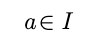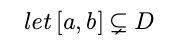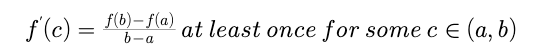# MAXIMA AND MINIMA , LOCAL MAXIMUM/ MINIMUM, GLOBAL MAXIMUM/ MINIMUM

MAXIMA AND MINIMA

LOCAL MAXIMA AND MINIMA:

let y = f(x) be a function defined at x = a and also in the vicinity of the point x = a, then f(x) is said to have a local maximum at x =a if the value of the function at x = a is greater than the value of the function at the neighboring point of x = a.

Similarly, f(x) is said to have a local minimum at x = a, if the value of the function at x = a is less than the value of the function at the neighboring points of x = a.

A TEST FOR LOCAL MAXIMUM/ MINIMUM

Test for local maximum/minimum at x= a if f(x) is differentiable at x = a.

Let f(x) is differentiable at x= a and it is a critical point of function f' (a) =0 and if f '(x) function changes its sign while passing through the point x = a then,

• f(x) would have a local maximum at x = a if f'(a - 0) > O and f’(a + 0) <0. It means that f'(x) should change its sign from positive to negative.

• f(x) would have a local minimum at x = a  if f'(a - 0) <O and f'(a + 0) > 0. It means that f'(x) should change its sign from negative to positive.

• If fix) doesn’t change its sign while passing through x = a then f(x) would have neither a maximum nor minimum at point x = a.

Second-order derivative test for maxima and minima

Let f(x) be a differentiable function on an interval I. letand f''(x) is continuous at x = a then

• x = a is a point of local maximum if f'(a) = 0 and f''(a) < 0.

• x = a is a point of local minimum if f'(a) = 0 and f''(a) > 0.

• if f'(a) = f''(a) and f'''(a) =! 0 if exists  then x = a  is neither a point of local maximum nor a point of local minimum.

Test for local ma ximum/minimum at x= a if f(x) is not differentiable at x = a:

When f(x) is continuous at x = a and f'(a-h) and f'(a + h) exist and are non-zero, then f(x) has a local maximum or minimum at x= a if  f'(a-h) and f'(a + h) are of opposite signs.

When f(x) is continuous and f‘(a-h) and f(a + h) exist but one of them is zero, we should compare the information about the existence of local maximum/minimum from the basic definition of local maximum/minimum.

If f (x) is not continuous at x= a and f'(a—h) and/or f'(a + h) are not finite  then compare the values of f(x) at the neighbouring points of x=a.

Concept of Global maximum and minimum

let y = f(x) be a given function with Domain D.Global maximum/minimum of f(x) in [a, b] is basically the greatest/least value of f(x) in [a, b]. Global maximum/minimum in [a, b] would always occure at critical points of f(x) with in [a, b] or at the end points of the interval.

Rolle’s Theorem

It states that if y = f(x) be a given function and satisfies the following conditions.

• f(x) be continuous in [a,b]

• f(x) be differentiable in (a, b)

• f(a) = f(b) then f'(c) =0 at least once for some c E (a, b)

Lagrange’s Mean Value Theorem

It states that if if y = f(x) be a given function and satisfies the following conditions

• f(x) be continuous in [a,b]

• f(x) be differentiable in (a, b)

thenPost By : Rahul Kumar 04 Jan, 2020 1557 views Maths